# Spatial Coverage Sampling with spcosa

## Introduction

The spcosa-package implements algorithms for

1. spatial coverage sampling and
2. random sampling from compact geographical strata.

These algorithms are based on the k-means algorithm (de Gruijter et al., 2006, Walvoort et al., 2010).

Spatial coverage sampling is known to be an efficient sampling method for model-based mapping (kriging). Random sampling from compact geographical strata is recommended for design-based estimation of spatial means, proportions, etc.

In this vignette, the usage of the package will be demonstrated by means of several examples.

## Initialisation

The spocsa-package can be attached by means of

library(spcosa)
Loading required package: rJava

In addition, we will also attach the ggplot2-package for modifying plots:

library(ggplot2)

Although the implemented optimisation algorithms are deterministic in nature, they use a user-specified number (nTry, see examples below) of random initial configurations to reduce the risk of ending up in an unfavourable local optimum. In order to be able to reproduce the sampling patterns at a later stage, the pseudo random number generator of R has to be initialised first:

set.seed(314)

The spcosa-package depends on the sp-package (Pebesma & Bivand, 2005) for storing spatial information, and the ggplot2-package (Wickham, 2009) for visualisation. A basic knowledge of the sp-package is highly recommended. Knowledge of the ggplot2-package is only needed for fine-tuning spcosa graphics. Consult the superb ggplot2-website for details and illustrative examples.

## Algorithms

The basic idea is to distribute sampling points evenly over the study area by selecting these points in compact spatial strata (a.k.a. geostrata). Compact strata can be constructed by k-means clustering of the points making up a fine grid representing the study area of interest. Two k-means algorithms have been implemented in the spcosa-package: a transfer algorithm and a swopping algorithm (Walvoort et al, 2010). The transfer algorithm obtains compact clusters (strata) by transferring cells from one cluster to the other, whereas the swopping algorithm achieves this by swopping cells between clusters. The first algorithm results in compact clusters, whereas the second algorithm results in compact clusters of equal size. For reasons of efficiency, both algorithms have been implemented in the Java language and communicate with R (R Core Team, 2015) by means of the rJava-package (Urbanek, 2013).

## Examples

In this section, the spcosa-package will be demonstrated by means of several examples.

### Spatial coverage sampling without prior points

First, a vector or raster representation of the study area has to be created that uses one of the spatial classes of the sp-package (Pebesma & Bivand, 2005). This can be accomplished by means of functions in the rgdal-package (Bivand et al., 2015). In this section, we simply create a grid programmatically.

library(sp)
grd <- expand.grid(s1 = 1:100, s2 = 1:50)
gridded(grd) <- ~ s1 * s2

To obtain a uniform distribution of sampling points over the study area, the sampling points will be selected at the centroids of compact spatial strata. Compact strata can be constructed by invoking the stratify method:

stratification <- stratify(grd, nStrata = 75, nTry = 10)

In this example, the study area has been partitioned into 75 compact strata. The resulting stratification can be plotted by means of:

plot(stratification)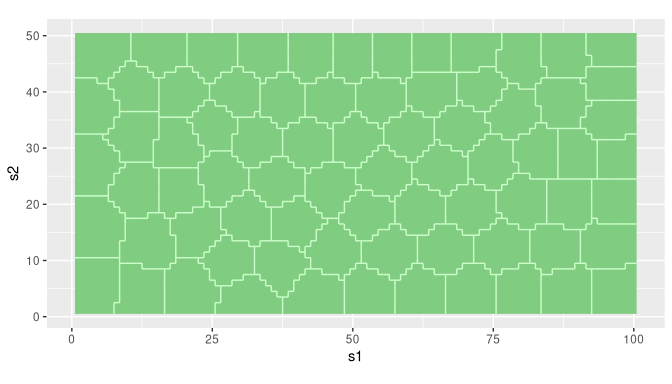Each plot can be modified by adding ggplot2-functions to the plot-method:

plot(stratification) +
scale_x_continuous(name = "Easting (km)") +
scale_y_continuous(name = "Northing (km)")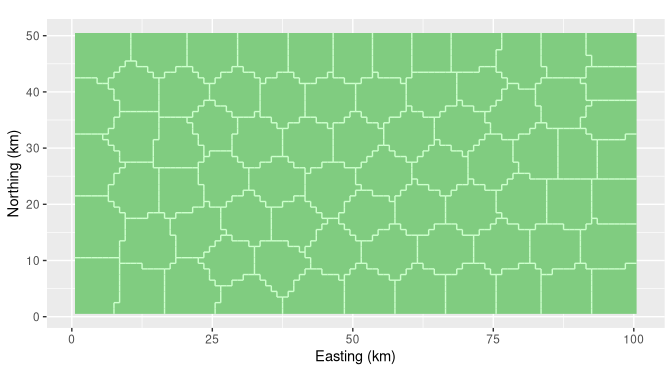The spsample-method, an overloaded method from the sp-package, can be used for selecting the centroid of each stratum:

sampling_pattern <- spsample(stratification)

The plot-method can be used to visualise the resulting sampling pattern:

plot(sampling_pattern)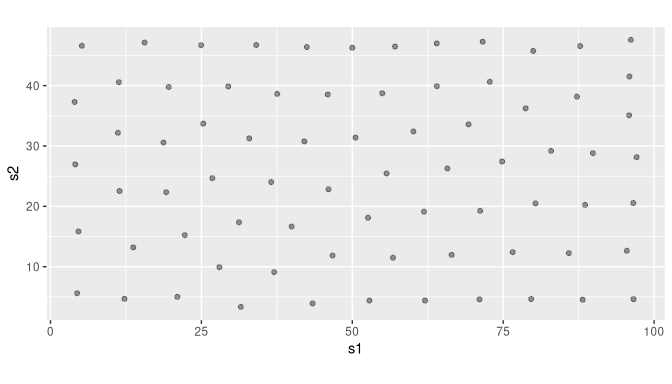The sampling pattern can also be plotted on top of the stratification:

plot(stratification, sampling_pattern)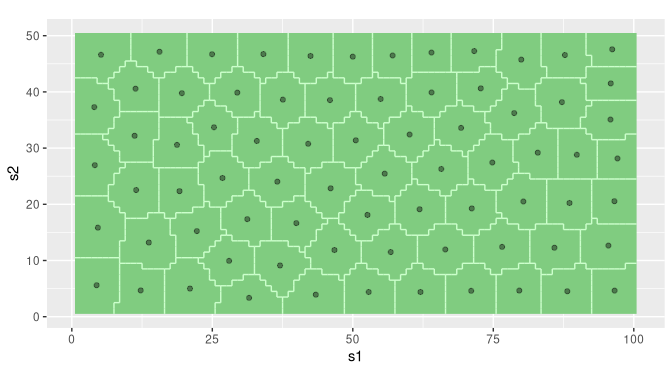The resulting sampling locations can be extracted by means of a simple type cast to class data.frame:

sampling_points <- as(sampling_pattern, "data.frame")
head(sampling_points)
         s1       s2
1 79.971831 45.74648
2 13.703704 13.19753
3 11.338710 40.56452
4 26.808824 24.67647
5  4.090909 26.96104
6 25.298246 33.70175

### Spatial coverage sampling with prior points

Sometimes, samples from previous sampling campaigns are available. In these situations, spatial infill sampling may be performed. This type of spatial coverage sampling aims to distribute new sampling points evenly over the study area, while taking the locations of existing sampling points into account. Suppose a data.frame is available containing the coordinates of 50 existing sampling points:

head(prior_points)
        s1         s2
1 45.27948 42.4586466
2 40.11544  7.1302025
3 10.18353  0.8628835
4 85.06315 25.0265617
5 86.31620 49.7829571
6 68.99990 36.4598513

Twenty-five new points can be assigned to sparsely sampled regions by means of:

coordinates(prior_points) <- ~ s1 * s2
stratification <- stratify(grd, priorPoints = prior_points, nStrata = 75, nTry = 100)
sampling_pattern <- spsample(stratification)

Note that the total number of strata, and therefore the total number of points, equals 50+25=75. In addition, also note that the nTry argument has been set to 100. The algorithm will now use 100 random starting configurations and keeps the best solution to reduce the risk of ending up in an unfavourable local optimum.

plot(stratification, sampling_pattern)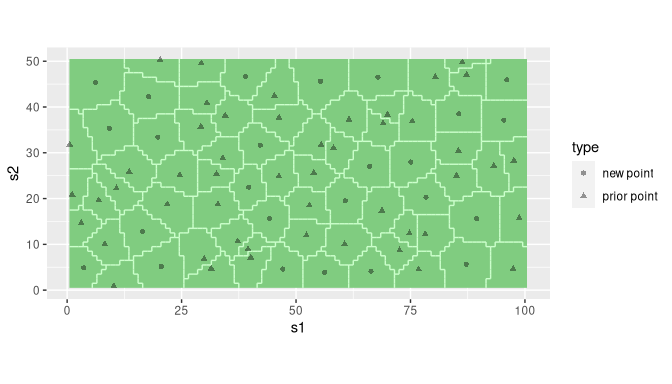Note that prior points and new points are represented by different symbols.

### Random sampling from compact geographical strata

In this section, the global mean clay and organic matter contents of the study area will be estimated by means of stratified simple random sampling. We will use grid grd (created in a previous section) as a representation of the study area. The study area will be partitioned into 25 compact strata:

stratification <- stratify(grd, nStrata = 25, nTry = 10)
plot(stratification)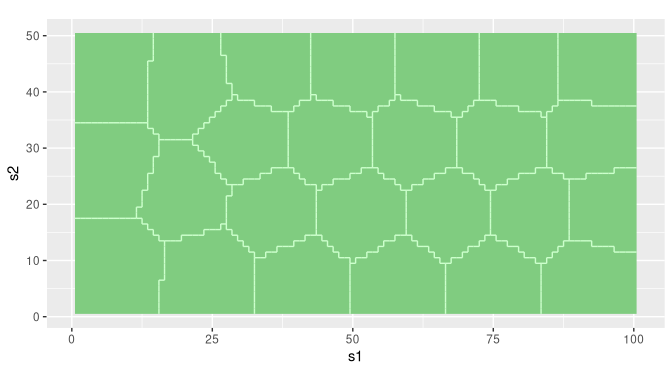The spsample-method can be used to randomly sample two sampling units per stratum:

sampling_pattern <- spsample(stratification, n = 2)
plot(stratification, sampling_pattern)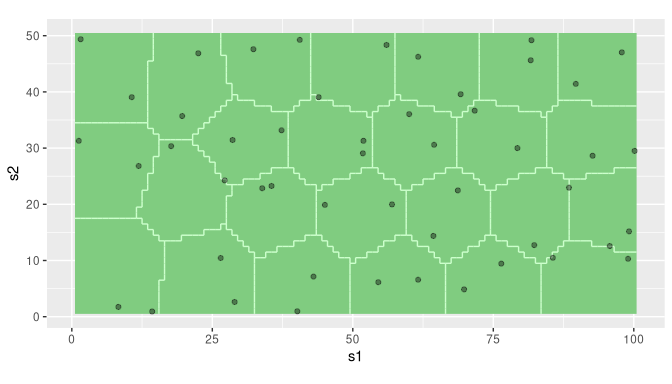Sampling points can be extracted by means of a type cast to class data.frame:

sampling_points <- as(sampling_pattern, "data.frame")
head(sampling_points)
           s1       s2
2234 33.88272 22.85582
2236 35.53852 23.26313
4841 40.56035 49.25627
4732 32.31102 47.60208
999  98.98614 10.30268
986  85.60128 10.48244

Next, some field and laboratory work has to be done. Suppose the resulting clay and SOM contents are stored in data.frame my_data (note that in this example we use simulated data):

my_data <- data.frame(clay = rbeta(50, 2, 25), SOM = rbeta(50, 1, 50))
head(my_data)
        clay         SOM
1 0.11155230 0.027001630
2 0.10683151 0.028900583
3 0.01279166 0.003675933
4 0.07658828 0.017279823
5 0.06358627 0.009849191
6 0.14557616 0.012373539

The spatial mean clay and soil organic matter contents can be estimated by (de Gruijter et al., 2006):

estimate("spatial mean", stratification, sampling_pattern, my_data)
      clay        SOM
0.07339864 0.01884442 

In estimating the spatial mean, differences in surface area of the strata are taken into account. Note, that the spatial mean is estimated for all columns in my_data. The standard error can be estimated in a similar way:

estimate("standard error", stratification, sampling_pattern, my_data)
       clay         SOM
0.006511187 0.003650515 

The spatial cumulative distribution function (SCDF) (see de Gruijter et al., 2006) can be estimated by means of

scdf <- estimate("scdf", stratification, sampling_pattern, my_data)

The SCDFs are returned as a list of matrices, i.e. one matrix for each property:

head(scdf$clay)  value cumFreq [1,] 0.01279166 0.0000 [2,] 0.01835631 0.0192 [3,] 0.01839286 0.0349 [4,] 0.02048009 0.0585 [5,] 0.02064662 0.0769 [6,] 0.02177574 0.0960 head(scdf$SOM)
            value cumFreq
[1,] 0.0003909092  0.0000
[2,] 0.0004350313  0.0195
[3,] 0.0010540480  0.0386
[4,] 0.0015136903  0.0579
[5,] 0.0023285072  0.0772
[6,] 0.0024394096  0.1028

The SCDFs for clay and SOM are visualised below.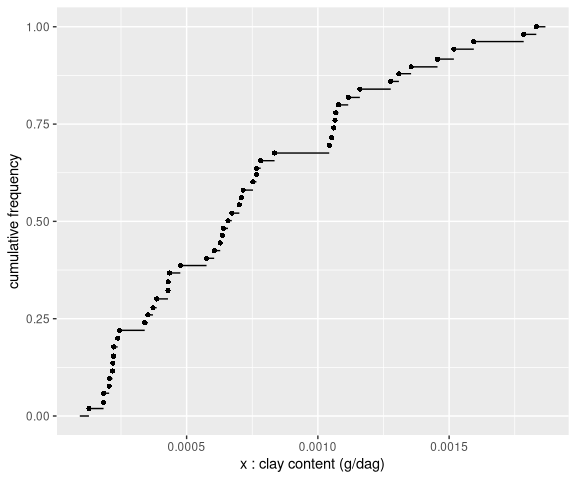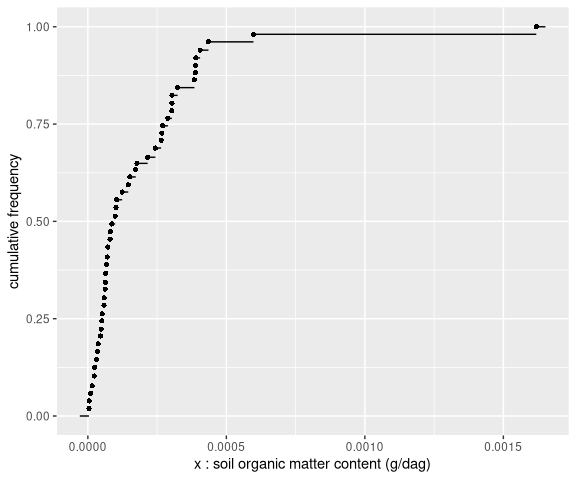### Stratified simple random sampling for composites

In this example, the aim is to estimate the global mean clay and organic matter contents of a field. To reduce laboratory costs, the soil aliquots collected at the sampling locations will be bulked into composite samples. The study area is a field near the village of Farmsum, in the North-East of the Netherlands. An ESRI shape file of this field is available in the maps directory of the spcosa-package. It can be loaded by means the readOGR-function in the rgdal package (Bivand et al., 2015):

library(rgdal)
Please note that rgdal will be retired by the end of 2023,
plan transition to sf/stars/terra functions using GDAL and PROJ
at your earliest convenience.

rgdal: version: 1.5-32, (SVN revision 1176)
Geospatial Data Abstraction Library extensions to R successfully loaded
Loaded GDAL runtime: GDAL 3.5.1, released 2022/06/30
Path to GDAL shared files: /usr/share/gdal
GDAL does not use iconv for recoding strings.
GDAL binary built with GEOS: TRUE
Loaded PROJ runtime: Rel. 9.0.1, June 15th, 2022, [PJ_VERSION: 901]
Path to PROJ shared files: /home/dennis/.local/share/proj:/usr/share/proj
PROJ CDN enabled: FALSE
Linking to sp version:1.5-0
To mute warnings of possible GDAL/OSR exportToProj4() degradation,
use options("rgdal_show_exportToProj4_warnings"="none") before loading sp or rgdal.
directory <- system.file("maps", package = "spcosa")
shp_farmsum <- readOGR(dsn = directory, layer = "farmsum", verbose = FALSE)

First, the field will be stratified into, say, 20 compact strata of equal size. Strata of equal size are desirable to simplify fieldwork, i.e. equal supports of soil can be collected at the sampling locations (Brus et al., 1999, de Gruijter et al., 2006).

stratification <- stratify(shp_farmsum, nStrata = 20, equalArea = TRUE, nTry = 10)
plot(stratification)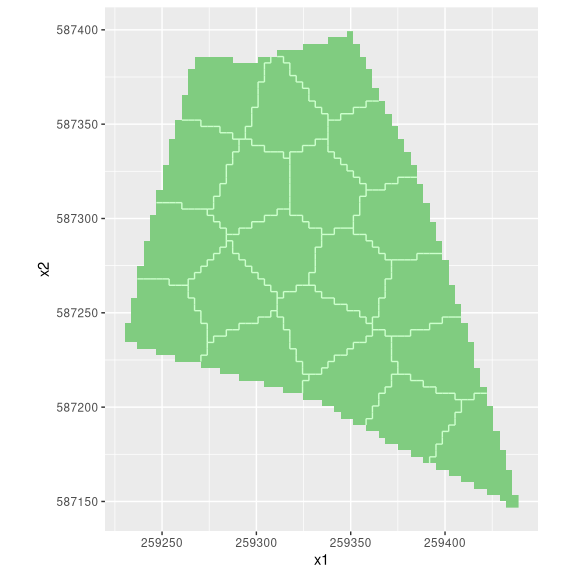Next, two sampling units will be selected at random in each stratum. At least two sampling units per stratum are required to estimate the sampling variance of the estimated mean.

sampling_pattern <- spsample(stratification, n = 2, type = "composite")
plot(stratification, sampling_pattern)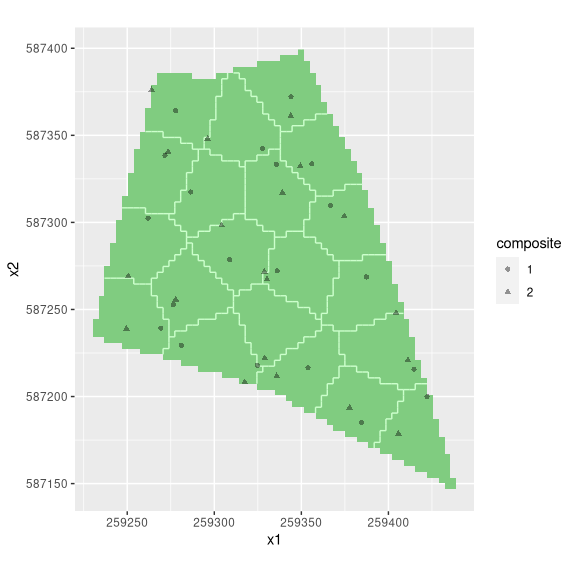Sampling points can be extracted means of:

sampling_points <- as(sampling_pattern, "data.frame")
head(sampling_points)
  composite       x1       x2
1         1 259387.4 587268.7
2         2 259404.4 587248.0
3         1 259262.0 587302.4
4         2 259250.6 587269.0
5         1 259414.7 587215.7
6         2 259411.2 587220.8

Note that an extra column has been added specifying the sampling units to be bulked into each composite. A composite sample is formed by bulking one aliquot (sampling unit) per stratum. Field work now results in a composite sample of size two. These data will be stored in a data.frame called my_data:

The spatial mean and its standard error can be estimated by means of:

estimate("spatial mean", stratification, sampling_pattern, my_data)
 clay   SOM
10.05  5.05 
estimate("standard error", stratification, sampling_pattern, my_data)
  clay    SOM
0.1225 0.0225 

If we do not want to bulk soil aliquots, the same stratification can be used to select a sample of 20 $$\times$$ 2 sampling locations:

sampling_pattern <- spsample(stratification, n = 2)
sampling_points <- as(sampling_pattern, "data.frame")
head(sampling_points)
        x1       x2
1 259368.8 587267.6
2 259389.6 587262.2
3 259261.1 587269.5
4 259249.3 587277.6
5 259403.0 587211.9
6 259377.9 587232.4
plot(stratification, sampling_pattern)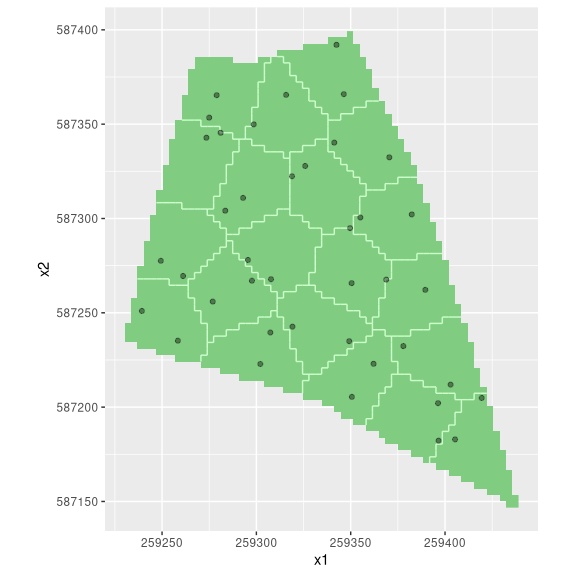## Special cases

### Map projections

When the projection attribute of a map is set to EPSG:4326 (lon/lat), great circle distances will be used for stratification. Otherwise, Euclidean distances will be used. At field scale, the differences between these distance measures is rather small. However, at continental and global scales, the projection attribute should be set to EPSG:4326.

To illustrate the effect of stratification on smaller spatial scales, consider two (relatively coarse) regular grids covering the surface of the earth:

grd <- expand.grid(
longitude = seq(from = -176, to = 180, by = 4),
latitude  = seq(from =  -86, to =  86, by = 4)
)
gridded(grd) <- ~ longitude * latitude

grd_crs <- grd
slot(grd_crs, "proj4string") <- CRS("EPSG:4326")

Note that grd is identical to grd_crs, except for projection attribute .

Both grids will be partitioned into 50 compact geographical strata:

strata     <- stratify(grd,     nStrata = 50)
strata_crs <- stratify(grd_crs, nStrata = 50)
plot(strata)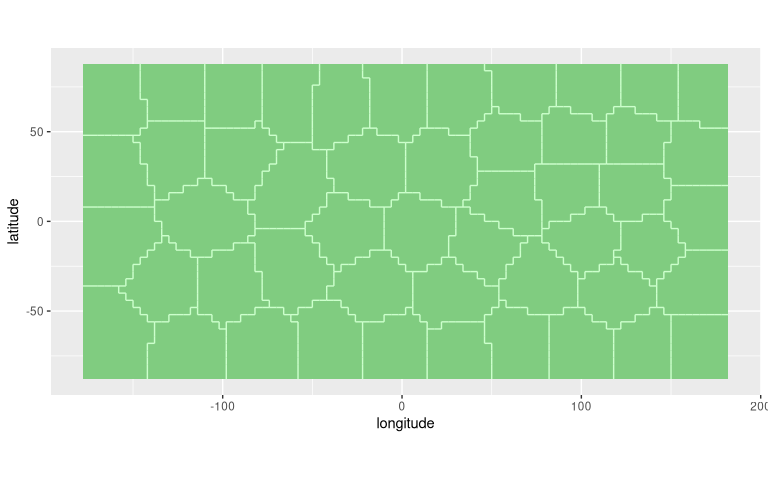plot(strata_crs)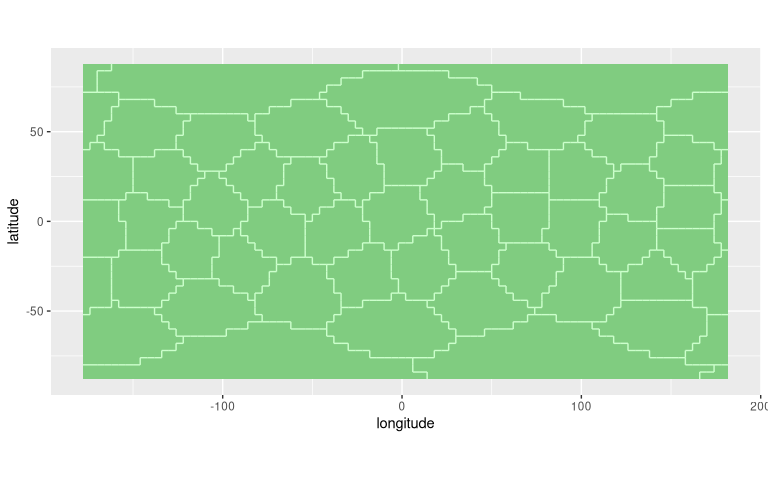Note that grd seems to have more compact strata near the geographic poles than grd_crs. However, the contrary is true. This becomes evident when both stratifications are projected on a sphere: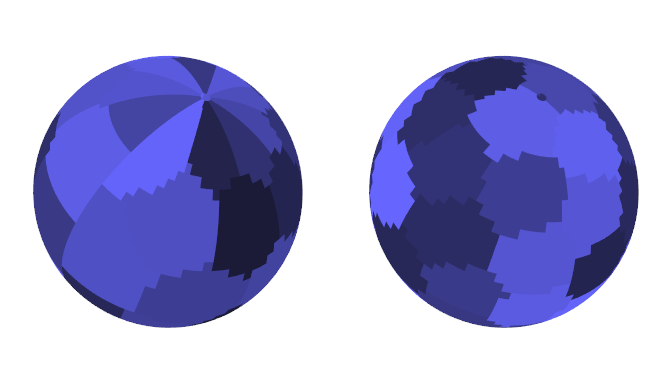The left figure is based on grd, and the right figure on grd_crs. The strata of grd_crs are clearly more compact than those of grd. In addition, grd suffers from pronounced edge effects near the poles and at 180 degrees longitude. The strata are discontinuous at this meridian, i.e., two points on opposite sides of the meridian are treated as very distant when squared Euclidean distances are used. The strata of grd_crs, on the other hand, have been optimised on a sphere by using squared great circle distances, and don’t suffer from edge effects, i.e., the great circle distance between two nearby points on opposite sides of this meridian is small.

### Simple random sampling

Although the spcosa-package is about sampling from compact strata, it can also be used for simple random sampling, by setting nStrata = 1:

shp_mijdrecht <- readOGR(
dsn = system.file("maps", package = "spcosa"),
layer = "mijdrecht", verbose = FALSE)
stratification <- stratify(shp_mijdrecht, nStrata = 1, nGridCells = 5000)
sampling_pattern <- spsample(stratification, n = 30)
plot(stratification, sampling_pattern)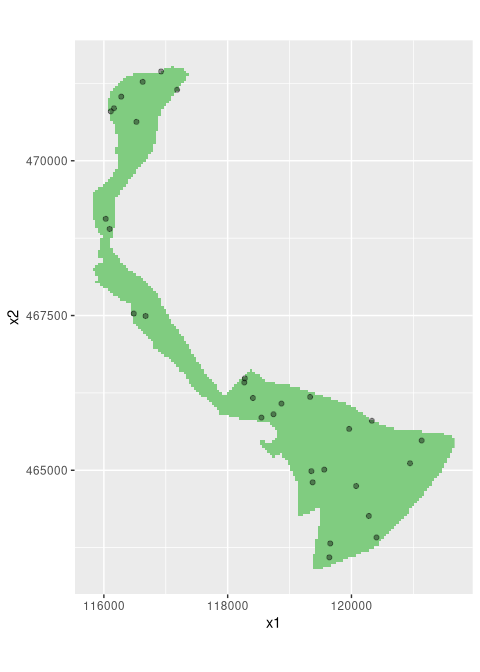### Sampling of non-convex areas

In case of spatial coverage sampling, sampling the centroid of each cluster may become problematic in case of non-convex areas. A centroid may be situated well outside the area of interest. If this happens, the sampling point will be relocated to the nearest grid cell that is part of the target universe. This pragmatic solution usually gives reasonable results. However, in some extreme situations the solution may be less desirable. As an example, consider the ‘doughnut’-shaped field below.

doughnut <- expand.grid(s1 = -25:25, s2 = -25:25)
d <- with(doughnut, sqrt(s1^2 + s2^2))
doughnut <- doughnut[(d < 25) & (d > 15), ]
coordinates(doughnut) <- ~ s1 * s2
gridded(doughnut) <- TRUE
stratification <- stratify(doughnut, nStrata = 2, nTry = 100)
sampling_pattern <- spsample(stratification)
plot(stratification, sampling_pattern)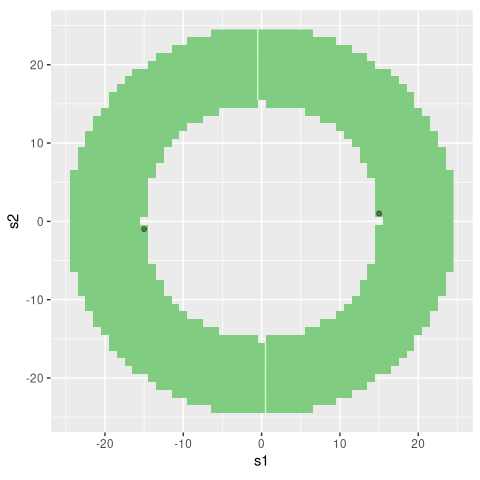Note that this problem does not arise in random sampling from compact geographical strata.

## Session information

R version 4.2.1 (2022-06-23)
Platform: x86_64-pc-linux-gnu (64-bit)
Running under: Arch Linux

Matrix products: default
BLAS:   /usr/lib/libblas.so.3.10.1
LAPACK: /usr/lib/liblapack.so.3.10.1

locale:
 LC_CTYPE=en_US.UTF-8          LC_NUMERIC=C
 LC_TIME=en_US.UTF-8           LC_COLLATE=C
 LC_MONETARY=en_US.UTF-8       LC_MESSAGES=en_US.UTF-8
 LC_PAPER=en_US.UTF-8          LC_NAME=en_US.UTF-8
 LC_MEASUREMENT=en_US.UTF-8    LC_IDENTIFICATION=en_US.UTF-8

attached base packages:
 stats     graphics  grDevices utils     datasets  methods   base

other attached packages:
 rgdal_1.5-32  sp_1.5-0      ggplot2_3.3.6 spcosa_0.4-1  rJava_1.0-6

loaded via a namespace (and not attached):
 highr_0.9        bslib_0.4.0      compiler_4.2.1   pillar_1.8.0
 jquerylib_0.1.4  tools_4.2.1      digest_0.6.29    lattice_0.20-45
 jsonlite_1.8.0   evaluate_0.16    lifecycle_1.0.1  tibble_3.1.8
 gtable_0.3.0     pkgconfig_2.0.3  rlang_1.0.4      DBI_1.1.3
 cli_3.3.0        rstudioapi_0.13  yaml_2.3.5       xfun_0.32
 fastmap_1.1.0    withr_2.5.0      dplyr_1.0.9      stringr_1.4.0
 knitr_1.39       generics_0.1.3   sass_0.4.2       vctrs_0.4.1
 tidyselect_1.1.2 grid_4.2.1       glue_1.6.2       R6_2.5.1
 fansi_1.0.3      rmarkdown_2.14   farver_2.1.1     purrr_0.3.4
 magrittr_2.0.3   scales_1.2.0     htmltools_0.5.3  assertthat_0.2.1
 colorspace_2.0-3 labeling_0.4.2   utf8_1.2.2       stringi_1.7.8
 munsell_0.5.0    cachem_1.0.6